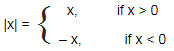# Simplification

## Important points to Remember:

• 1) This chapter is very important because numerical on simplification are based on concepts related decimal fraction, square and cube roots, H.C.F. and L.C.M., numbers, etc.
• 2) How to find H.C.F. and L.C.M.
• 3) Tricks to solve decimal fractions
• 4) Number system, types of numbers
• 5) How to find square root and cube root

### Basic Formulae: (Must Remember)

• 1) (a - b)2 = (a2 + b2 - 2ab)
• 2) (a + b)2 = (a2 + b2 + 2ab)
• 3) (a + b) (a – b) = (a2 – b2 )
• 4) (a3 + b3) = (a + b) (a2 – ab + b2)
• 5) (a3 - b3) = (a - b) (a2 – ab + b2)
• 6) (a + b + c)2 = a2 + b2 + c2 + 2 (ab + bc + ca)
• 7) (a3 + b3 + c3 – 3abc) = (a + b + c) (a2 + b2 + c2 – ab – bc – ac)## Quick Tips and Tricks

### 1) Virnaculum (Bar ‘¬¬–’):

If a given expression contains a virnaculum (bar), then first simplify the given expression under virnaculum and then follow the rule of‘BODMAS’.

Step1: When simplifying the given expressions, first brackets are to be removed in the order: ‘––’, ‘( )’, ‘{ }’, ‘[ ]’

Step2: The operations are to be performed strictly in the order: Division, Multiplication, Addition and Subtraction

### 2) ‘BODMAS Rule’:

This rule is used to solve and find out the value of given expressions by performing the operations in a correct sequence.

BODMAS is the shortcut used to remember the procedure of simplification.

• B: Bracket
• O: Order (i.e. power, square root etc.)
• D: Division (Left to right)
• M: Multiplication (Left to right)
• A: Addition (Left to right)
• S: Subtraction (Left to right)

Step1: When simplifying the given expressions, first brackets are to be removed in the order: ‘––’, ‘( )’, ‘{ }’, ‘[ ]’

Step2: The operations are to be performed strictly in the order: Division, Multiplication, Addition and Subtraction

### 3) Modulus of a real number

The modulus of a real number or the absolute value is defined as follows: Modulus of x is written as |x|### About us

EntryTest.com is a free service for students seeking successful career.CAT - College of Admission Tests. All rights reserved. College of Admission Tests Online Test Preparation The CAT Online